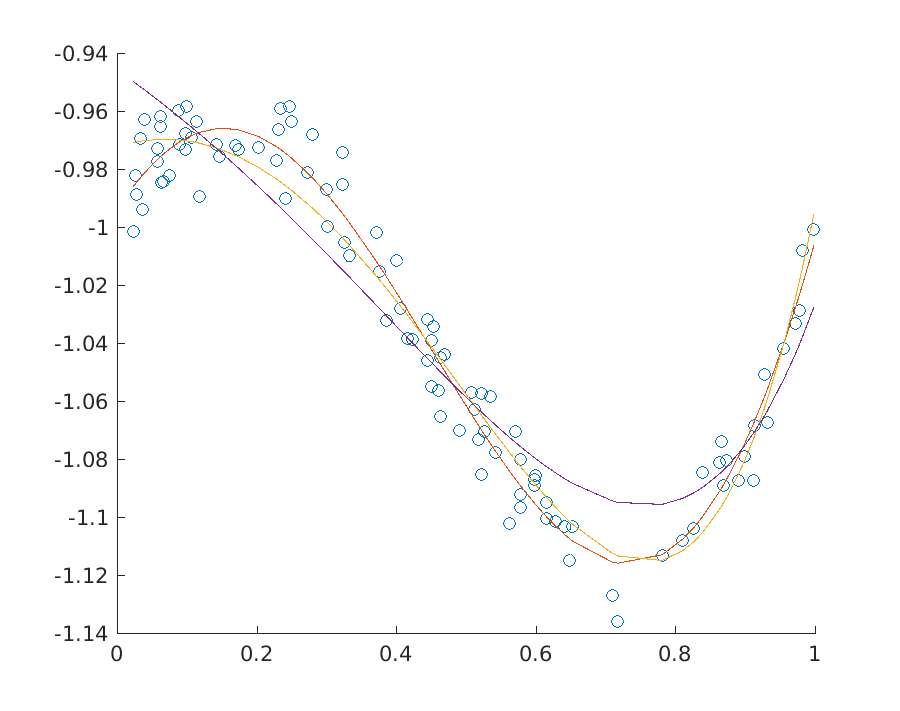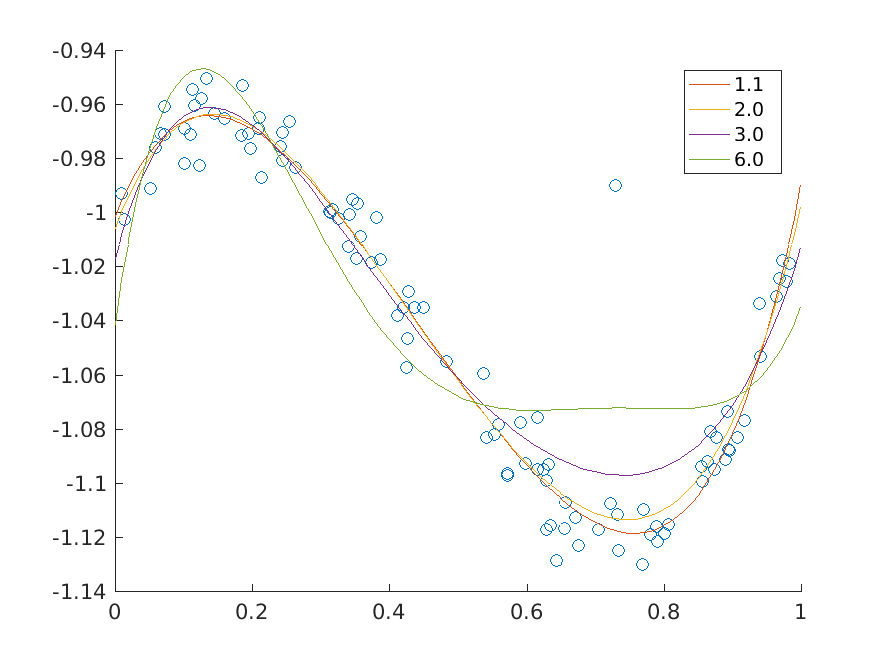# 11.2 Least Squares and Other Norm Minimization Problems¶

A frequently occurring problem in statistics and in many other areas of science is a norm minimization problem

(11.13)$\begin{split}\begin{array}{ll} \mbox{minimize} & \|F x - g\|,\\ \mbox{subject to} & Ax=b, \end{array}\end{split}$

where $$x\in\real^n$$ and of course we can allow other types of constraints. The objective can involve various norms: infinity norm, 1-norm, 2-norm, $$p$$-norms and so on. For instance the most popular case of the 2-norm corresponds to the least squares linear regression, since it is equivalent to minimization of $$\|Fx-g\|_2^2$$.

## 11.2.1 Least squares, 2-norm¶

In the case of the 2-norm we specify the problem directly in conic quadratic form

(11.14)$\begin{split}\begin{array}{ll} \mbox{minimize} & t,\\ \mbox{subject to} & (t, Fx-g)\in\Q^{k+1}, \\ & Ax=b. \end{array}\end{split}$

The first constraint of the problem can be represented as an affine conic constraint. This leads to the following model.

Listing 11.8 Script solving problem (11.14) Click here to download.
% Least squares regression
% minimize \|Fx-g\|_2
function x = norm_lse(F,g,A,b)
clear prob;
[r, res] = mosekopt('symbcon echo(0)');
n = size(F,2);
k = size(g,1);
m = size(A,1);

% Linear constraints in [x; t]
prob.a   = [A, zeros(m,1)];
prob.buc = b;
prob.blc = b;
prob.blx = -inf*ones(n+1,1);
prob.bux = inf*ones(n+1,1);
prob.c   = [zeros(n,1); 1];

% Affine conic constraint
prob.f = sparse([zeros(1,n), 1; F, zeros(k,1)]);
prob.g = [0; -g];
prob.accs = [ res.symbcon.MSK_DOMAIN_QUADRATIC_CONE k+1 ];

% Solve
[r, res] = mosekopt('minimize echo(0)', prob);
x = res.sol.itr.xx(1:n);
end


## 11.2.2 Ridge regularisation¶

Regularisation is classically applied to reduce the impact of outliers and to control overfitting. In the conic version of ridge (Tychonov) regression we consider the problem

(11.15)$\begin{split}\begin{array}{ll} \mbox{minimize} & \|F x - g\|_2+ \gamma\|x\|_2,\\ \mbox{subject to} & Ax=b, \end{array}\end{split}$

which can be written explicitly as

(11.16)$\begin{split}\begin{array}{ll} \mbox{minimize} & t_1+\gamma t_2,\\ \mbox{subject to} & (t_1, Fx-g)\in\Q^{k+1}, \\ & (t_2, x)\in\Q^{n+1},\\ & Ax=b. \end{array}\end{split}$

The implementation is a small extension of that from the previous section.

Listing 11.9 Script solving problem (11.16) Click here to download.
% Least squares regression with regularization
% minimize \|Fx-g\|_2 + gamma*\|x\|_2
function x = norm_lse_reg(F,g,A,b,gamma)
clear prob;
[r, res] = mosekopt('symbcon echo(0)');
n = size(F,2);
k = size(g,1);
m = size(A,1);

% Linear constraints in [x; t1; t2]
prob.a   = [A, zeros(m,2)];
prob.buc = b;
prob.blc = b;
prob.blx = -inf*ones(n+2,1);
prob.bux = inf*ones(n+2,1);
prob.c   = [zeros(n,1); 1; gamma];

% Affine conic constraint
prob.f = sparse([zeros(1,n),        1, 0; ...
F,           zeros(k,2); ...
zeros(1,n),        0, 1; ...
eye(n),      zeros(n,2) ]);
prob.g = [0; -g; zeros(n+1,1)];

% Solve
[r, res] = mosekopt('minimize echo(0)', prob);
x = res.sol.itr.xx(1:n);
end


Note that classically least squares problems are formulated as quadratic problems and then the objective function would be written as

$\|Fx-g\|_2^2+\gamma\|x\|_2^2.$

This version can easily be obtained by replacing the quadratic cone with an appropriate rotated quadratic cone in (11.16). Then they core of the implementation would change as follows:

Listing 11.10 Script solving classical quadratic ridge regression Click here to download.
prob.f = sparse([zeros(1,n),        1, 0; ...
zeros(1,n+2)           ; ...
F,           zeros(k,2); ...
zeros(1,n),        0, 1; ...
zeros(1,n+2)           ; ...
eye(n),      zeros(n,2) ]);
prob.g = [0; 0.5; -g; 0; 0.5; zeros(n,1)];


Fig. 11.2 shows the solution to a polynomial fitting problem for a few variants of least squares regression with and without ridge regularization.Fig. 11.2 Three fits to a dataset at various levels of regularization.¶

## 11.2.3 Lasso regularization¶

In lasso or least absolute shrinkage and selection operator the regularization term is the 1-norm of the solution

(11.17)$\begin{split}\begin{array}{ll} \mbox{minimize} & \|F x - g\|_2+ \gamma\|x\|_1,\\ \mbox{subject to} & Ax=b. \end{array}\end{split}$

This variant typically tends to give preference to sparser solutions, i.e. solutions where only a few elements of $$x$$ are nonzero, and therefore it is used as an efficient approximation to the cardinality constrained problem with an upper bound on the 0-norm of $$x$$. To see how it works we first implement (11.17) adding the constraint $$t\geq \|x\|_1$$ as a series of linear constraints

$u_i\geq -x_i,\ u_i\geq x_i,\ t\geq \sum u_i,$

so that eventually the problem becomes

$\begin{split}\begin{array}{ll} \mbox{minimize} & t_1+\gamma t_2,\\ \mbox{subject to} & u+x\geq 0,\\ & u-x\geq 0,\\ & t_2-e^Tu \geq 0,\\ & Ax=b,\\ & (t_1, Fx-g) \in \Q^{k+1}. \end{array}\end{split}$
Listing 11.11 Script solving problem (11.17) Click here to download.
% Least squares regression with lasso regularization
% minimize \|Fx-g\|_2 + gamma*\|x\|_1
function x = norm_lse_lasso(F,g,A,b,gamma)
clear prob;
[r, res] = mosekopt('symbcon echo(0)');
n = size(F,2);
k = size(g,1);
m = size(A,1);

% Linear constraints in [x; u; t1; t2]
prob.a   = [A,         zeros(m,n+2)      ; ...
eye(n),    eye(n), zeros(n,2); ...
-eye(n),   eye(n), zeros(n,2); ...
zeros(1,n) -ones(1,n), 0, 1 ];
prob.buc = [b; inf*ones(2*n+1,1)];
prob.blc = [b; zeros(2*n+1,1)];
prob.blx = -inf*ones(2*n+2,1);
prob.bux = inf*ones(2*n+2,1);
prob.c   = [zeros(2*n,1); 1; gamma];

% Affine conic constraint
prob.f = sparse([zeros(1,2*n), 1, 0; F, zeros(k,n+2)]);
prob.g = [0; -g];
prob.accs = [ res.symbcon.MSK_DOMAIN_QUADRATIC_CONE k+1 ];

% Solve
[r, res] = mosekopt('minimize echo(0)', prob);
x = res.sol.itr.xx(1:n);
end


The sparsity pattern of the solution of a large random regression problem can look for example as follows:

Lasso regularization
Gamma 0.0100  density 99%   |Fx-g|_2: 54.3722
Gamma 0.1000  density 87%   |Fx-g|_2: 54.3939
Gamma 0.3000  density 67%   |Fx-g|_2: 54.5319
Gamma 0.6000  density 40%   |Fx-g|_2: 54.8379
Gamma 0.9000  density 26%   |Fx-g|_2: 55.0720
Gamma 1.3000  density 12%   |Fx-g|_2: 55.1903


## 11.2.4 p-norm minimization¶

Now we consider the minimization of the $$p$$-norm defined for $$p>1$$ as

(11.18)$\|y\|_p = \left(\sum_i |y_i|^p \right)^{1/p}.$

We have the optimization problem:

(11.19)$\begin{split}\begin{array}{ll} \mbox{minimize} & \|F x - g\|_p,\\ \mbox{subject to} & Ax=b. \end{array}\end{split}$

Increasing the value of $$p$$ forces stronger penalization of outliers as ultimately, when $$p\to\infty$$, the $$p$$-norm $$\|y\|_p$$ converges to the infinity norm $$\|y\|_\infty$$ of $$y$$. According to the Modeling Cookbook the $$p$$-norm bound $$t\geq \|Fx-g\|_p$$ can be added to the model using a sequence of three-dimensional power cones and we obtain an equivalent problem

(11.20)$\begin{split}\begin{array}{ll} \mbox{minimize} & t \\ \mbox{subject to} & (r_i,t,(Fx-g)_i)\in\POW_3^{1/p,1-1/p}, \\ & e^Tr = t, \\ & Ax = b. \end{array}\end{split}$

The power cones can be added one by one to the structure representing affine conic constraints. Each power cone will require one $$r_i$$, one copy of $$t$$ and one row from $$F$$ and $$g$$. An alternative solution is to create the vector

$[r_1;\ldots;r_k;t;\ldots;t;Fx-g]$

and then reshuffle its elements into

$[r_1;t;F_1x-g_1;\ldots;r_k;t;F_kx-g_k]$

using an appropriate permutation matrix. This approach is demonstrated in the code below.

Listing 11.12 Script solving problem (11.20) Click here to download.
% P-norm minimization
% minimize \|Fx-g\|_p
function x = norm_p_norm(F,g,A,b,p)
clear prob;
[r, res] = mosekopt('symbcon echo(0)');
n = size(F,2);
k = size(g,1);
m = size(A,1);

% Linear constraints in [x; r; t]
prob.a   = [A, zeros(m,k+1); zeros(1,n), ones(1,k), -1];
prob.buc = [b; 0];
prob.blc = [b; 0];
prob.blx = -inf*ones(n+k+1,1);
prob.bux = inf*ones(n+k+1,1);
prob.c   = [zeros(n+k,1); 1];

% Permutation matrix which picks triples (r_i, t, F_ix-g_i)
M = [];
for i=1:3
M = [M, sparse(i:3:3*k, 1:k, ones(k,1), 3*k, k)];
end

% Affine conic constraint
prob.f = M * sparse([zeros(k,n), eye(k), zeros(k,1); zeros(k,n+k), ones(k,1); F, zeros(k,k+1)]);
prob.g = M * [zeros(2*k,1); -g];
prob.accs = [ repmat([res.symbcon.MSK_DOMAIN_PRIMAL_POWER_CONE, 3, 2, 1.0, p-1], 1, k) ];

% Solve
[r, res] = mosekopt('minimize echo(0)', prob);
x = res.sol.itr.xx(1:n);
endFig. 11.3 $$p$$-norm minimizing fits of a polynomial of degree at most 5 to the data for various values of $$p$$.¶